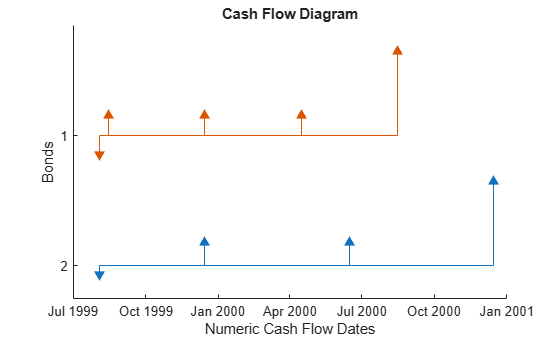# cfport

Portfolio form of cash flow amounts

## Syntax

``[CFBondDate,AllDates,AllTF,IndByBond] = cfport(CFlowAmounts,CFlowDates)``
``[CFBondDate,AllDates,AllTF,IndByBond] = cfport(___,TFactors)``

## Description

example

````[CFBondDate,AllDates,AllTF,IndByBond] = cfport(CFlowAmounts,CFlowDates)` computes a vector of all cash flow dates of a bond portfolio, and a matrix mapping the cash flows of each bond to those dates. Use the matrix for pricing the bonds against a curve of discount factors.```

example

````[CFBondDate,AllDates,AllTF,IndByBond] = cfport(___,TFactors)` specifies options using one or more optional arguments in addition to the input arguments in the previous syntax.```

## Examples

collapse all

Use the function `cfamounts` to calculate the cash flow amounts, cash flow dates, and time factors for each of two bonds. Then use the function `cfplot` to plot the cash flow diagram.

```Settle = '03-Aug-1999'; Maturity = ['15-Aug-2000';'15-Dec-2000']; CouponRate= [0.06; 0.05]; Period = [3;2]; Basis = [1;0]; [CFlowAmounts, CFlowDates, TFactors] = cfamounts(CouponRate,... Settle, Maturity, Period, Basis); cfplot(CFlowDates,CFlowAmounts) xlabel('Numeric Cash Flow Dates') ylabel('Bonds') title('Cash Flow Diagram')```Call the function `cfport` to map the cash flow amounts to the cash flow dates. Each row in the resultant `CFBondDate` matrix represents a bond. Each column represents a date on which one or more of the bonds has a cash flow. A 0 means the bond did not have a cash flow on that date. The dates associated with the columns are listed in `AllDates`. For example, the first bond had a cash flow of `2.000` on `730347`. The second bond had no cash flow on this date For each bond, `IndByBond` indicates the columns of `CFBondDate`, or dates in `AllDates`, for which a bond has a cash flow.

```[CFBondDate, AllDates, AllTF, IndByBond] = ... cfport(CFlowAmounts, CFlowDates, TFactors)```
```CFBondDate = 2×7 -1.8000 2.0000 2.0000 2.0000 0 102.0000 0 -0.6694 0 2.5000 0 2.5000 0 102.5000 ```
```AllDates = 7×1 730335 730347 730469 730591 730652 730713 730835 ```
```AllTF = 7×1 0 0.0663 0.7322 1.3989 1.7322 2.0663 2.7322 ```
```IndByBond = 2×5 1 2 3 4 6 1 3 5 7 NaN ```

Use the function `cfamounts` to calculate the cash flow amounts, cash flow dates, and time factors for each of two bonds.

```Settle = datetime(1999,8,3); Maturity = [datetime(2000,8,15) ; datetime(2000,12,15)]; CouponRate= [0.06; 0.05]; Period = [3;2]; Basis = [1;0]; [CFlowAmounts, CFlowDates, TFactors] = cfamounts(CouponRate,... Settle, Maturity, Period, Basis);```

Call the function `cfport` to map the cash flow amounts to the cash flow dates. Each row in the resultant `CFBondDate` matrix represents a bond. Each column represents a date on which one or more of the bonds has a cash flow. A `0` means the bond did not have a cash flow on that date. The dates associated with the columns are listed in `AllDates` returned as a datetime array.

```[CFBondDate, AllDates, AllTF, IndByBond] = ... cfport(CFlowAmounts, CFlowDates, TFactors)```
```CFBondDate = 2×7 -1.8000 2.0000 2.0000 2.0000 0 102.0000 0 -0.6694 0 2.5000 0 2.5000 0 102.5000 ```
```AllDates = 7x1 datetime 03-Aug-1999 15-Aug-1999 15-Dec-1999 15-Apr-2000 15-Jun-2000 15-Aug-2000 15-Dec-2000 ```
```AllTF = 7×1 0 0.0663 0.7322 1.3989 1.7322 2.0663 2.7322 ```
```IndByBond = 2×5 1 2 3 4 6 1 3 5 7 NaN ```

## Input Arguments

collapse all

Cash flow amounts, specified as number of bonds (`NUMBONDS`) by number of cash flows (`NUMCFS`) matrix with entries listing cash flow amounts corresponding to each date in `CFlowDates`.

Data Types: `double`

Cash flow dates, specified as an `NUMBONDS`-by-`NUMCFS` matrix with rows listing cash flow dates using a datetime array, string array, or date character vectors for each bond and padded with `NaN`s. If `CFlowDates` is a serial date number or a date character vector, `AllDates` is returned as an array of serial date numbers. If `CFlowDates` is a datetime array, then `AllDates` is returned as a datetime array.

To support existing code, `cfport` also accepts serial date numbers as inputs, but they are not recommended.

Data Types: `char` | `string` | `datetime`

(Optional) Time between settlement and the cash flow date, specified as an `NUMBONDS`-by-`NUMCFS` matrix with entries listing the time between settlement and the cash flow date measured in semiannual coupon periods.

Data Types: `double`

## Output Arguments

collapse all

Cash flows indexed by bond and by date, returned as an `NUMBONDS` by number of dates (`NUMDATES`) matrix. Each row contains a bond's cash flow values at the indices corresponding to entries in `AllDates`. Other indices in the row contain zeros.

List of all dates that have any cash flow from the bond portfolio, returned as an `NUMDATES`-by-`1` matrix. The `AllDates` matrix is expressed in datetime format (if `CFlowDates` is in datetime format).

Time factors corresponding to the dates in `AllDates`, returned as an `NUMDATES`-by-`1` matrix. If `TFactors` is not entered, `AllTF` contains the number of days from the first date in `AllDates`.

Indices by bond, returned as an `NUMBONDS`-by-`NUMCFS` matrix. The ith row contains a list of indices into `AllDates` where the ith bond has cash flows. Since some bonds have more cash flows than others, the matrix is padded with `NaN`s.

## Version History

Introduced before R2006a

expand all